#The 2nd grade math games on our website focus on several critical math areas such as
the understanding of base-ten notation and place value, building fluency with addition and subtraction, using simple concepts of multiplication, measuring length with appropriate units, finding the perimeter, classifying and analyzing shapes, telling time, and counting back change in coins and bills.

In some games students will analyze and interpret data using bar graphs and Venn diagrams. Play these fun math games and become stronger in math before you know it!Balance the Addition Equation Math Racing Game

In this fast-paced math racing game, 2nd Grade students must work quickly to give the numbers that make the addition equations correct.2nd Grade Halloween Math Game
This online Halloween math game is engaging, fun, and get kids excited about learning. Students will practice comparing three-digit numbers, telling time to the nearest five minutes, and solving problems with money. For each correct answer, kids will get a chance to destroy monsters and score points.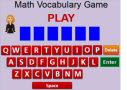2nd Grade Math Vocabulary Game

In this fun and interactive vocabulary game, young students will review important math vocabulary terms and difinitions.Balance the Subtraction Equation Math Racing Game

Work quickly to give the numbers that make both sides of the subtraction equations equal in this Balance the Subtraction Equation Math Racing Game.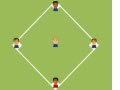Make-it-Correct Addition Baseball Game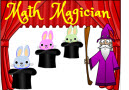Math Magician Add Two-Digit Numbers Game

Show how well you can add two-digit numbers by playing this fun Math Magician Addition Game.Add Doubles Math Racing Game

Have lots of fun playing this Add Doubles Math Racing Game as you add numbers and their doubles to successfully cross the finish line.Add Three Numbers Halloween Math Game
Play this colorful halloween math game to practice adding three numbers up to 20.Make-it-Correct Subtraction Baseball Game
Have a great time learning subtraction by playing this exciting Baseball Math Make-it-Correct Subtraction Game.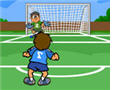In this exciting game, kids will practice adding two-digit whole numbers. For each correct answer, they will get a chance to kick the ball and score points.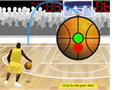Subtracting Two-Digit Numbers

In this interactive soccer game, 2nd grade students will practice adding 2-digit numbers.

Multiplication Concentration Games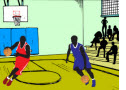Mixed Operations Basketball Game

Apply the correct order of operations and perform different operations in this interactive two-player game.Matching Time Game

For each question, kids will identify the correct time to the nearest 5 minutes by matching the analog clocks with the correct digital clocks.Money Word Search

Play a fun and interactive online word search game about money.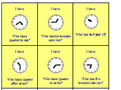Who has? I have!
This is a printable time game that can be used as a classroom activity with elementary students.Multiplication Game

In this fast-paced car racing game, 2nd and 3rd grade students will practice multiplying one-digit numbers.Basketball Multiplication Game

Kids will have a lot of fun playing this interactive basketball game, but they will also practice multiplying one-digit whole numbers.Short Division Basketball Game